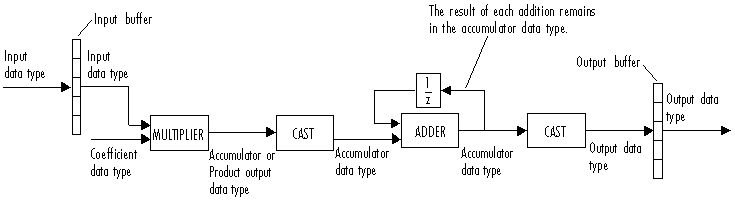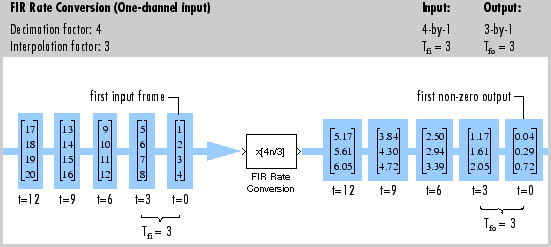# FIR Rate Conversion

Upsample, filter, and downsample input signals

## Library

Filtering / Multirate Filters

`dspmlti4`## Description

The FIR Rate Conversion block resamples the discrete-time input such that its sample period is K/L times the input sample period (Tsi). K is the integer value you specify for the Decimation factor parameter, and L is the integer value you specify for the Interpolation factor parameter.

The block treats each column of the input as a separate channel, and resamples the data in each channel independently over time. To do so, the block implements a polyphase filter structure and performs the following operations:

1. Upsamples the input to a higher rate by inserting L-`1` zeros between input samples.

2. Passes the upsampled data through a direct-form II transpose FIR filter.

3. Downsamples the filtered data to a lower rate by discarding K-`1` consecutive samples following each sample that the block retains.

The polyphase filter implementation is more efficient than a straightforward upsample-filter-decimate algorithm. See Orfanidis  for more information.

### Specifying the Resampling Rate

You specify the resampling rate of the FIR Rate Conversion block using the Decimation factor and Interpolation factor parameters. For an Mi-by-N matrix input, the Decimation factor, K, and the Interpolation factor, L, must satisfy the following requirements:

• K and L must be relatively prime integers; that is, the ratio K/L cannot be reduced to a ratio of smaller integers.

• $\frac{K}{L}=\frac{{M}_{i}}{{M}_{o}}$, where Mi and Mo are the integer frame sizes of the input and output, respectively.

You can satisfy the second requirement by setting the Decimation factor, K, equal to the input frame size, Mi. When you do so, the output frame size, Mo, equals the Interpolation factor, L.

By changing the frame size in this way, the block is able to hold the frame period constant (Tfi = Tfo) and achieve the desired conversion of the sample period, such that

`${T}_{so}=\frac{K}{L}×{T}_{si}$`

where Tso is the output sample period.

### Specifying the FIR Filter Coefficients

To specify the filter coefficients, select the mode you want the FIR Rate Conversion block to operate in. Select the mode in the Coefficient source group box.

• Dialog parameters — Enter information about the filter, such as coefficients, in the block dialog box.

• Filter object — Specify the filter using a `dsp.FIRRateConverter` System object™.

• Auto (default) — Choose the filter coefficients automatically.

When you select the Dialog parameters option, you use the FIR filter coefficients parameter to specify the numerator coefficients of the FIR filter transfer function H(z).

`$H\left(z\right)=B\left(z\right)={b}_{1}+{b}_{2}{z}^{-1}+\dots +{b}_{m}{z}^{-\left(m-1\right)}$`

You can generate the FIR filter coefficient vector, ```[b(1) b(2) ... b(m)]```, using one of the DSP System Toolbox™ filter design functions such as `designMultirateFIR`, `firnyquist`, `firhalfband`, `firgr` or `firceqrip`.

The coefficient vector you specify must have a length greater than the interpolation factor (m>L). The FIR filter must be a lowpass filter with a normalized cutoff frequency, no greater than `min`(`1`/L,1/K). The block internally initializes all filter states to zero.

When you select Auto, the block designs an FIR multirate filter with the decimation factor specified in Decimation factor and interpolation factor specified in Interpolation factor. The `designMultirateFIR` function designs the filter and returns the coefficients used by the block. For more information on the filter design, see Orfanidis .

### Fixed-Point Data Types

The following diagram shows the data types used within the FIR Rate Conversion block for fixed-point signals.You can set the coefficient, product output, accumulator, and output data types in the block dialog box as discussed in Dialog Box. The diagram shows that input data is stored in the input buffer in the same data type and scaling as the input. Filtered data resides in the output buffer in the output data type and scaling that you set in the block dialog. The block stores any initial conditions in the output buffer using the output data type and scaling that you set in the block dialog box.

The output of the multiplier is in the product output data type when at least one of the inputs to the multiplier is real. When both of the inputs to the multiplier are complex, the result of the multiplication is in the accumulator data type. For details on the complex multiplication performed, see Multiplication Data Types.

### Note

When the block input is fixed point, all internal data types are signed fixed point.

## Examples

### Example 1

The following figure shows how the FIR Rate Conversion block converts a `4`-by-`1` input with a sample period of `3`/`4`, to a `3`-by-`1` output with a sample period of `1`. The frame period (Tf) of 3 remains constant.### Example 2

The `ex_audio_frc` model provides a simple illustration of one way to convert a speech signal from one sample rate to another. In this model, the data is first sampled at 22,050 Hz and then resampled at 8000 Hz. If you listen to the output, you can hear that the high frequency content has been removed from the signal, although the speech sounds basically the same.

## Dialog Box

### Coefficient Source

The FIR Rate Conversion block can operate in three different modes. Select the mode in the Coefficient source group box.

• Dialog parameters — Enter information about the filter, such as coefficients, in the block mask.

• Filter object — Specify the filter using a `dsp.FIRRateConverter` System object.

• Auto (default) — Choose the filter coefficients automatically.

Different items appear on the FIR Rate Conversion block dialog box depending on whether you select Dialog parameters, Filter object, or Auto in the Coefficient source group box.

## References

 Orfanidis, Sophocles J. Introduction to Signal Processing. Upper Saddle River, NJ: Prentice-Hall, 1996.

## Supported Data Types

PortSupported Data Types

Input

• Double-precision floating point

• Single-precision floating point

• Fixed point

• 8-, 16-, and 32-bit signed integers

• 8-, 16-, and 32-bit unsigned integers

Output

• Double-precision floating point

• Single-precision floating point

• Fixed point

• 8-, 16-, and 32-bit signed integers

• 8-, 16-, and 32-bit unsigned integers

 Downsample DSP System Toolbox Upsample DSP System Toolbox FIR Decimation DSP System Toolbox FIR Interpolation DSP System Toolbox CIC Decimation DSP System Toolbox CIC Interpolation DSP System Toolbox FIR Halfband Interpolator DSP System Toolbox FIR Halfband Decimator DSP System Toolbox IIR Halfband Interpolator DSP System Toolbox IIR Halfband Decimator DSP System Toolbox CIC Compensation Interpolator DSP System Toolbox CIC Compensation Decimator DSP System Toolbox `dsp.CICCompensationDecimator` DSP System Toolbox `dsp.CICCompensationInterpolator` DSP System Toolbox `dsp.FIRHalfbandDecimator` DSP System Toolbox `dsp.FIRHalfbandInterpolator` DSP System Toolbox `dsp.FIRDecimator` DSP System Toolbox `dsp.FIRInterpolator` DSP System Toolbox `firnyquist` DSP System Toolbox `firhalfband` DSP System Toolbox `firgr` DSP System Toolbox `firceqrip` DSP System Toolbox

See the following sections for related information:

Watch now